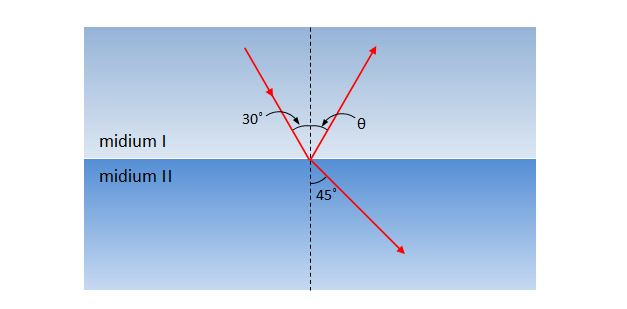# Refraction of a waveRefraction is the change in the direction of a wave due to a change in its transmission medium. The above diagram shows this phenomenon schematically, where a monochromatic light with wavelength $\lambda$ is passing from medium I to medium II. At the boundary surface between the two mediums, some portion of the light is reflected and the rest is refracted with $45^\circ$ refraction angle. Which of the following statements is NOT correct?

a) The reflection angle $\theta$ is $30^\circ.$
b) The frequency of the monochromatic light in medium I is greater than that in medium II.
c) The speed of the monochromatic light in medium I is faster than that in medium II.

×